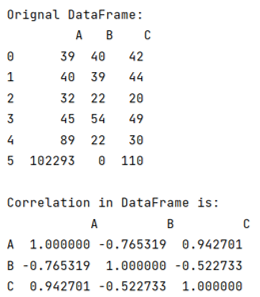# How to use corr() to get the correlation between two columns?

By using the corr() method, learn how to get the correlation between two columns?
Submitted by Pranit Sharma, on June 18, 2022

Pandas is a special tool that allows us to perform complex manipulations of data effectively and efficiently. Inside pandas, we mostly deal with a dataset in the form of DataFrame. DataFrames are 2-dimensional data structures in pandas. DataFrames consist of rows, columns, and the data.

There is always some kind of similarity/difference between all the values of all the columns in pandas DataFrame. This similarity or difference is known as the correlation of values in a DataFrame. To find the correlation in pandas, we use pandas.DataFrame.corr() method in pandas.

## pandas.DataFrame.corr() Method

This method is used to find the pair-wise correlation (similarities / differences) of the column values. The important point is if there is any null value present in any column, pandas.DataFrame.corr() automatically excludes it and also the non-numeric data is ignored.

Syntax:

```DataFrame.corr(
method='pearson',
min_periods=1
)
```

To work with pandas, we need to import pandas package first, below is the syntax:

```import pandas as pd
```

Let us understand with the help of an example,

## Python code to get the correlation between two columns

```# Importing pandas package
import pandas as pd

# Importing seaborn package
import seaborn as sn

# Import matplotlib package
import matplotlib.pyplot as plt

# Create a DataFrame
df = pd.DataFrame({
'A':[39,40,32,45,89,102293],
'B':[40,39,22,54,22,0],
'C':[42,44,20,49,30,110]
})

# Display original DataFrame
print("Original DataFrame:\n",df,"\n")

# Finding correlation
result = df.corr(method ='pearson')

# Display result
print("Correlation in DataFrame is:\n",result,"\n")
```

Output:What's New (MCQs)

Top Interview Coding Problems/Challenges!

IncludeHelp's Blogs

Languages: » C » C++ » C++ STL » Java » Data Structure » C#.Net » Android » Kotlin » SQL
Web Technologies: » PHP » Python » JavaScript » CSS » Ajax » Node.js » Web programming/HTML
Solved programs: » C » C++ » DS » Java » C#
Aptitude que. & ans.: » C » C++ » Java » DBMS
Interview que. & ans.: » C » Embedded C » Java » SEO » HR
CS Subjects: » CS Basics » O.S. » Networks » DBMS » Embedded Systems » Cloud Computing
» Machine learning » CS Organizations » Linux » DOS
More: » Articles » Puzzles » News/Updates